# Mirrors and LensesPage 5

#### WATCH ALL SLIDES

The equations can be used for both converging and diverging lenses

Slide 34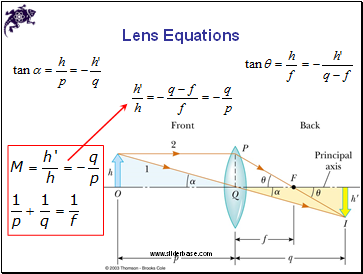Lens Equations

Slide 35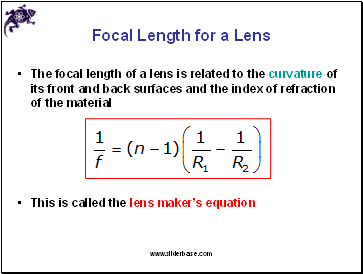## Focal Length for a Lens

The focal length of a lens is related to the curvature of its front and back surfaces and the index of refraction of the material

This is called the lens maker’s equation

Slide 36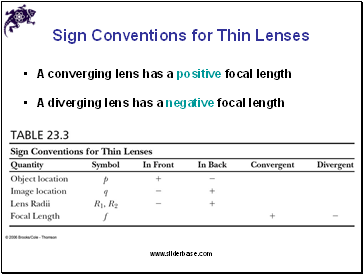## Sign Conventions for Thin Lenses

A converging lens has a positive focal length

A diverging lens has a negative focal length

Slide 37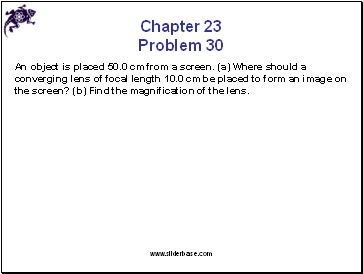Chapter 23 Problem 30

An object is placed 50.0 cm from a screen. (a) Where should a converging lens of focal length 10.0 cm be placed to form an image on the screen? (b) Find the magnification of the lens.

Slide 38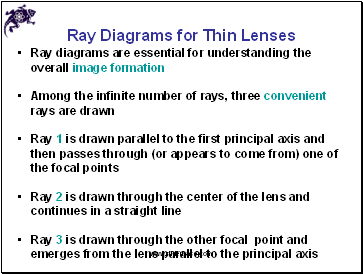## Ray Diagrams for Thin Lenses

Ray diagrams are essential for understanding the overall image formation

Among the infinite number of rays, three convenient rays are drawn

Ray 1 is drawn parallel to the first principal axis and then passes through (or appears to come from) one of the focal points

Ray 2 is drawn through the center of the lens and continues in a straight line

Ray 3 is drawn through the other focal point and emerges from the lens parallel to the principal axis

Slide 39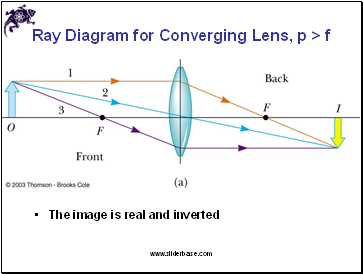Ray Diagram for Converging Lens, p > f

The image is real and inverted

Slide 40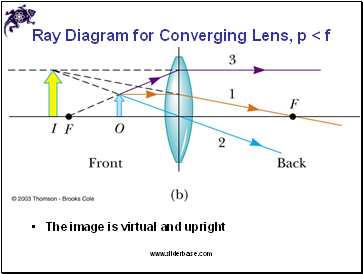Ray Diagram for Converging Lens, p < f

The image is virtual and upright

Slide 41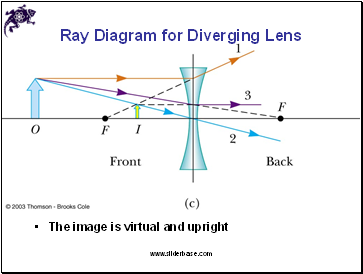Ray Diagram for Diverging Lens

The image is virtual and upright

Slide 42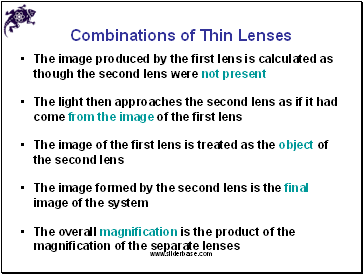## Combinations of Thin Lenses

The image produced by the first lens is calculated as though the second lens were not present

The light then approaches the second lens as if it had come from the image of the first lens

The image of the first lens is treated as the object of the second lens

The image formed by the second lens is the final image of the system

The overall magnification is the product of the magnification of the separate lenses

Slide 43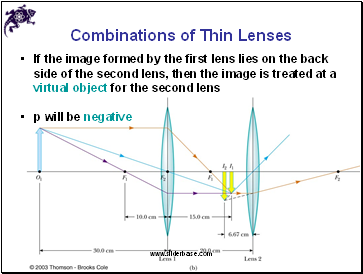Combinations of Thin Lenses

If the image formed by the first lens lies on the back side of the second lens, then the image is treated at a virtual object for the second lens

Go to page:
1  2  3  4  5  6  7